# Quantum Mechanics AP Physics B Quantum Quantum mechanics

• Slides: 19Quantum Mechanics AP Physics BQuantum? Quantum mechanics is the study of processes which occur at the atomic scale. The word "quantum" is derived From Latin to mean BUNDLE. Therefore, we are studying the motion of objects that come in small bundles called quanta. These tiny bundles that we are referring to are electrons traveling around the nucleus.“Newton, forgive me. . ”, Albert At the atomic scale Newtonian Mechanics Einstein cannot seem to describe the motion of particles. An electron trajectory between two points for example IS NOT a perfect parabolic trajectory as Newton's Laws predicts. Where Newton's Laws end Quantum Mechanics takes over. . . IN A BIG WAY! One of the most popular concepts concerning Quantum Mechanics is called , “The Photoelectric Effect”. In 1905, Albert Einstein published this theory for which he won the Nobel Prize in 1921.What is the Photoelectric Effect? In very basic terms, it is when electrons are released from a certain type of metal upon receiving enough energy from incident light. So basically, light comes down and strikes the metal. If the energy of the light wave is sufficient, the electron will then shoot out of the metal with some velocity and kinetic energy.The Electron-Volt = ENERGY Before we begin to discuss the photoelectric effect, we must introduce a new type of unit. Recall: This is a very useful unit as it shortens our calculations and allows us to stray away from using exponents.The Photoelectric Effect "When light strikes a material, electrons are emitted. The radiant energy supplies the work necessary to free the electrons from the surface. "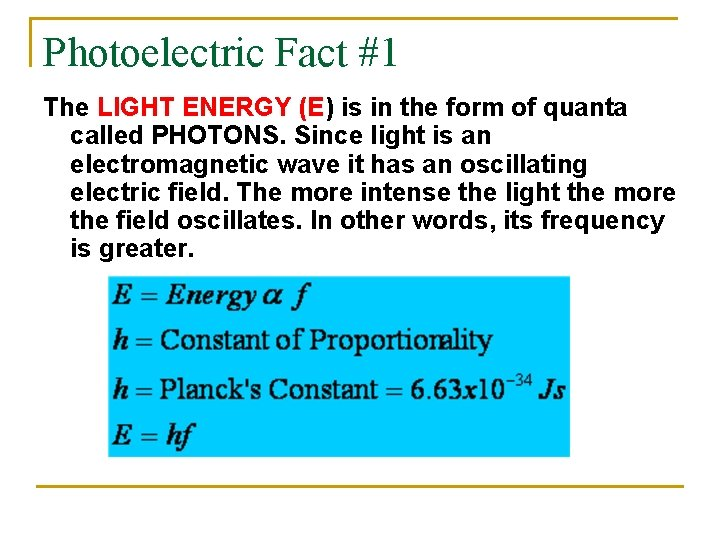Photoelectric Fact #1 The LIGHT ENERGY (E) is in the form of quanta called PHOTONS. Since light is an electromagnetic wave it has an oscillating electric field. The more intense the light the more the field oscillates. In other words, its frequency is greater.Light ReviewMore on Fact #1 Make sure you USE the correct constant! h 6. 63 x 10 -34 Js 4. 14 x 10 -15 e. Vs hc 1. 99 x 10 -25 Jm 1. 24 x 103 e. Vnm Planck’s Constant is the SLOPE of an Energy vs. Frequency graph!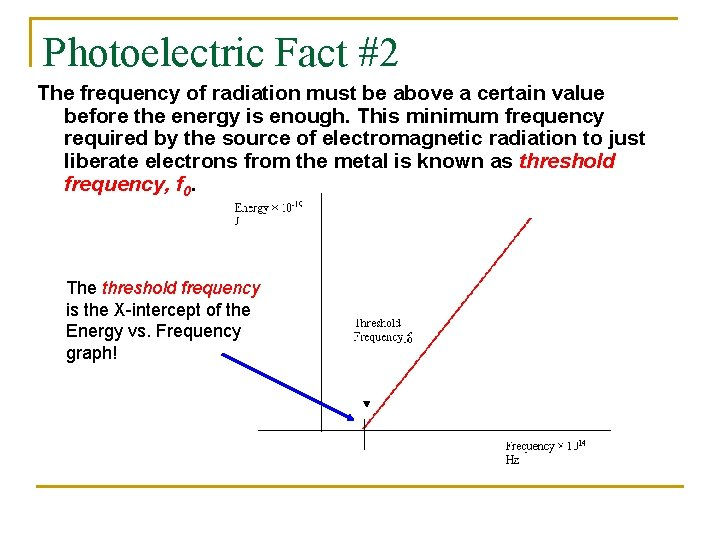Photoelectric Fact #2 The frequency of radiation must be above a certain value before the energy is enough. This minimum frequency required by the source of electromagnetic radiation to just liberate electrons from the metal is known as threshold frequency, f 0. The threshold frequency is the X-intercept of the Energy vs. Frequency graph!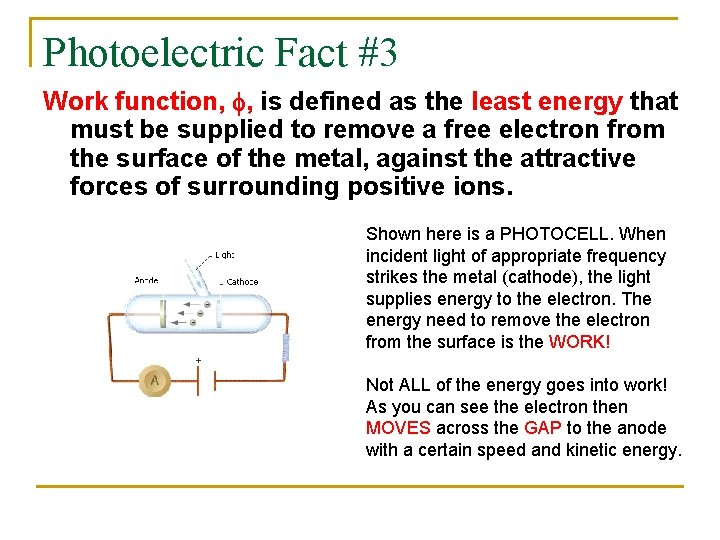Photoelectric Fact #3 Work function, f, is defined as the least energy that must be supplied to remove a free electron from the surface of the metal, against the attractive forces of surrounding positive ions. Shown here is a PHOTOCELL. When incident light of appropriate frequency strikes the metal (cathode), the light supplies energy to the electron. The energy need to remove the electron from the surface is the WORK! Not ALL of the energy goes into work! As you can see the electron then MOVES across the GAP to the anode with a certain speed and kinetic energy.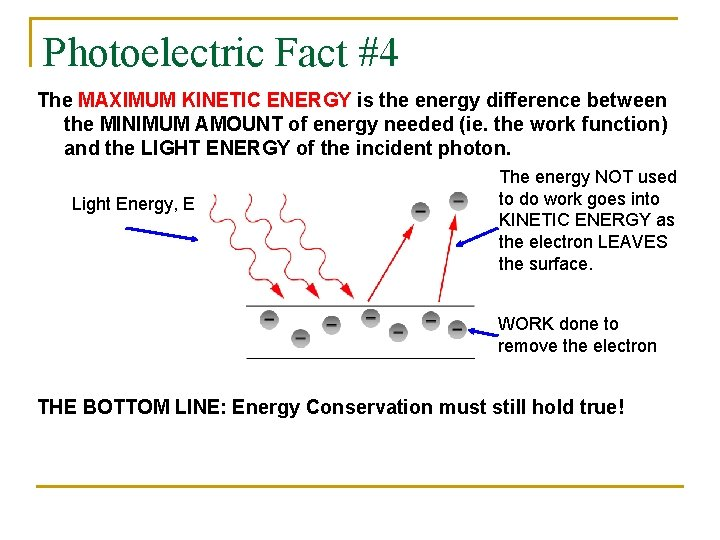Photoelectric Fact #4 The MAXIMUM KINETIC ENERGY is the energy difference between the MINIMUM AMOUNT of energy needed (ie. the work function) and the LIGHT ENERGY of the incident photon. Light Energy, E The energy NOT used to do work goes into KINETIC ENERGY as the electron LEAVES the surface. WORK done to remove the electron THE BOTTOM LINE: Energy Conservation must still hold true!Putting it all together KINETIC ENERGY can be plotted on the y axis and FREQUENCY on the xaxis. The WORK FUNCTION is the y – intercept as the THRESHOLD FREQUNECY is the x intercept. PLANCK‘S CONSTANT is the slope of the graph.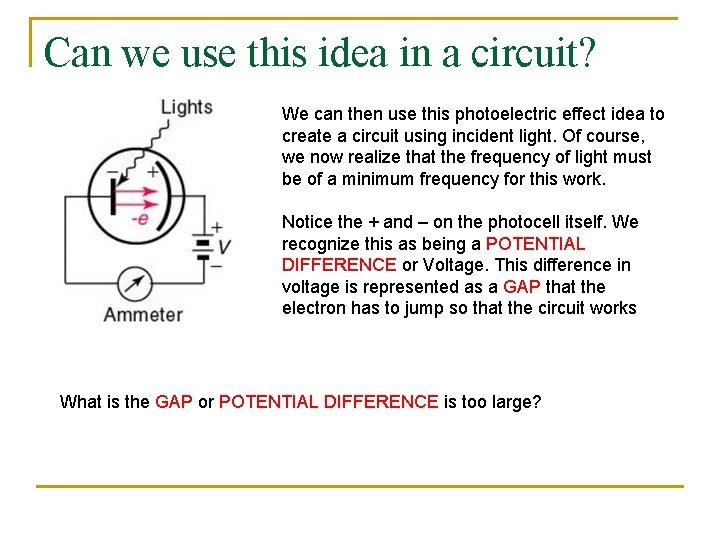Can we use this idea in a circuit? We can then use this photoelectric effect idea to create a circuit using incident light. Of course, we now realize that the frequency of light must be of a minimum frequency for this work. Notice the + and – on the photocell itself. We recognize this as being a POTENTIAL DIFFERENCE or Voltage. This difference in voltage is represented as a GAP that the electron has to jump so that the circuit works What is the GAP or POTENTIAL DIFFERENCE is too large?Photoelectric Fact #5 - Stopping Potential If the voltage is TOO LARGE the electrons WILL NOT have enough energy to jump the gap. We call this VOLTAGE point the STOPPING POTENTIAL. If the voltage exceeds this value, no photons will be emitted no matter how intense. Therefore it appears that the voltage has all the control over whether the photon will be emitted and thus has kinetic energy.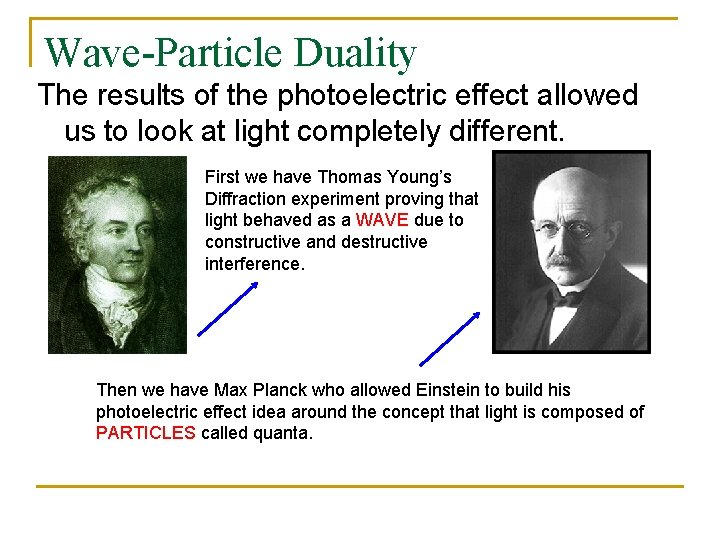Wave-Particle Duality The results of the photoelectric effect allowed us to look at light completely different. First we have Thomas Young’s Diffraction experiment proving that light behaved as a WAVE due to constructive and destructive interference. Then we have Max Planck who allowed Einstein to build his photoelectric effect idea around the concept that light is composed of PARTICLES called quanta.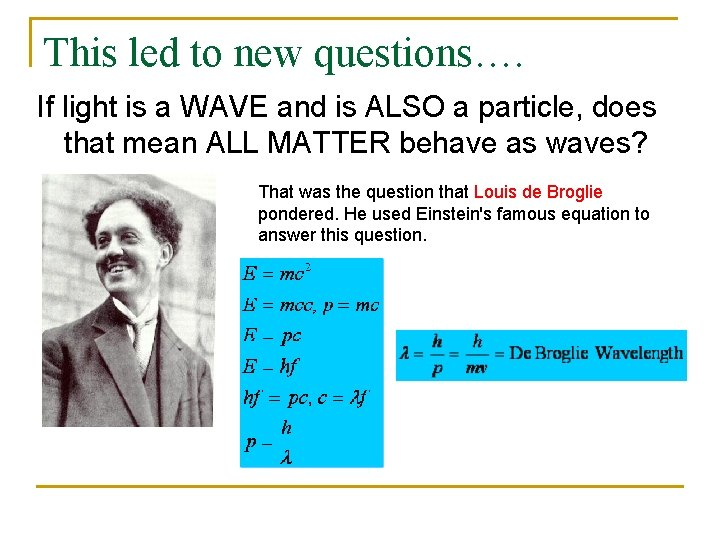This led to new questions…. If light is a WAVE and is ALSO a particle, does that mean ALL MATTER behave as waves? That was the question that Louis de Broglie pondered. He used Einstein's famous equation to answer this question.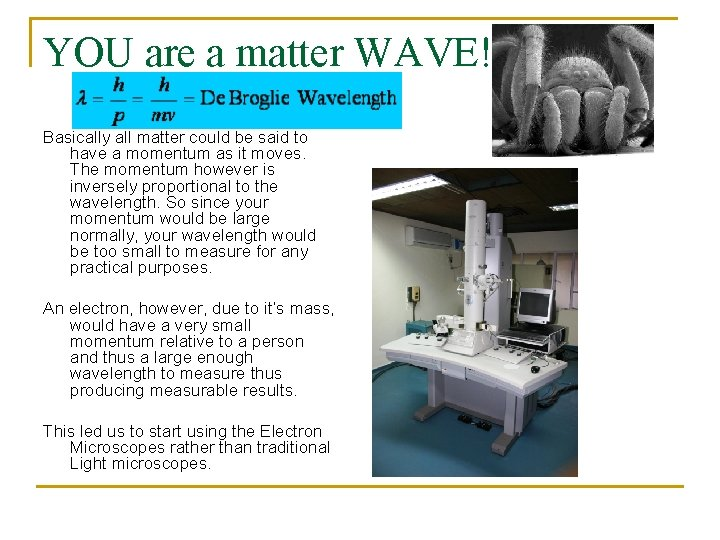YOU are a matter WAVE! Basically all matter could be said to have a momentum as it moves. The momentum however is inversely proportional to the wavelength. So since your momentum would be large normally, your wavelength would be too small to measure for any practical purposes. An electron, however, due to it’s mass, would have a very small momentum relative to a person and thus a large enough wavelength to measure thus producing measurable results. This led us to start using the Electron Microscopes rather than traditional Light microscopes.The electron microscope After the specimen is prepped. It is blasted by a bean of electrons. As the incident electrons strike the surface, electrons are released from the surface of the specimen. The de. Broglie wavelength of these released electrons vary in wavelength which can then be converted to a signal by which a 3 D picture can then be created based on the signals captured by the detector.